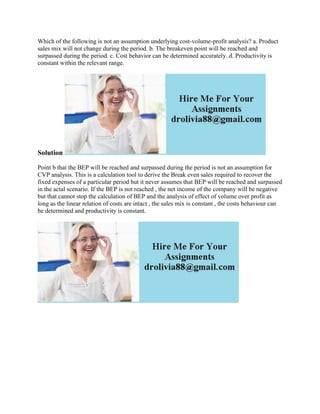Seu SlideShare está sendo baixado. ×

# Which of the following is not an assumption underlying cost-volume-pro.docx

Anúncio
Anúncio
Anúncio
Anúncio
Anúncio
Anúncio
Anúncio
Anúncio
Anúncio
Anúncio
AnúncioCarregando em…3
×

1 de 1 Anúncio

# Which of the following is not an assumption underlying cost-volume-pro.docx

Which of the following is not an assumption underlying cost-volume-profit analysis? a. Product sales mix will not change during the period. b. The breakeven point will be reached and surpassed during the period. c. Cost behavior can be determined accurately. d. Productivity is constant within the relevant range.
Solution
Point b that the BEP will be reached and surpassed during the period is not an assumption for CVP analysis. This is a calculation tool to derive the Break even sales required to recover the fixed expenses of a particular period but it never assumes that BEP will be reached and surpassed in the actal scenario. If the BEP is not reached , the net income of the company will be negative but that cannot stop the calculation of BEP and the analysis of effect of volume over profit as long as the linear relation of costs are intact , the sales mix is constant , the costs behaviour can be determined and productivity is constant.
.

Which of the following is not an assumption underlying cost-volume-profit analysis? a. Product sales mix will not change during the period. b. The breakeven point will be reached and surpassed during the period. c. Cost behavior can be determined accurately. d. Productivity is constant within the relevant range.
Solution
Point b that the BEP will be reached and surpassed during the period is not an assumption for CVP analysis. This is a calculation tool to derive the Break even sales required to recover the fixed expenses of a particular period but it never assumes that BEP will be reached and surpassed in the actal scenario. If the BEP is not reached , the net income of the company will be negative but that cannot stop the calculation of BEP and the analysis of effect of volume over profit as long as the linear relation of costs are intact , the sales mix is constant , the costs behaviour can be determined and productivity is constant.
.

Anúncio
Anúncio

### Which of the following is not an assumption underlying cost-volume-pro.docx

1. 1. Which of the following is not an assumption underlying cost-volume-profit analysis? a. Product sales mix will not change during the period. b. The breakeven point will be reached and surpassed during the period. c. Cost behavior can be determined accurately. d. Productivity is constant within the relevant range. Solution Point b that the BEP will be reached and surpassed during the period is not an assumption for CVP analysis. This is a calculation tool to derive the Break even sales required to recover the fixed expenses of a particular period but it never assumes that BEP will be reached and surpassed in the actal scenario. If the BEP is not reached , the net income of the company will be negative but that cannot stop the calculation of BEP and the analysis of effect of volume over profit as long as the linear relation of costs are intact , the sales mix is constant , the costs behaviour can be determined and productivity is constant.